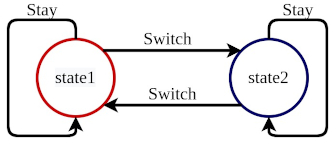## Coding a Simple Markov Decision Process

A Markov Decision Process (MDP) is a mathematical framework used to model decision-making situations where the outcome of a decision depends on both the current state of the system and the actions taken by the decision maker. In an MDP, the decision maker is represented as an agent, and the system is represented as a …